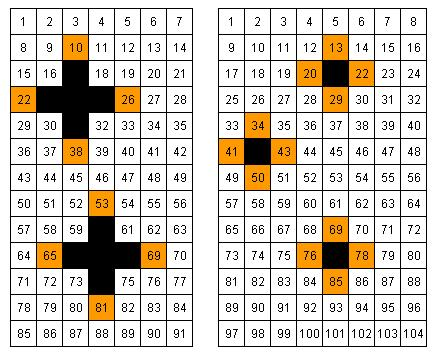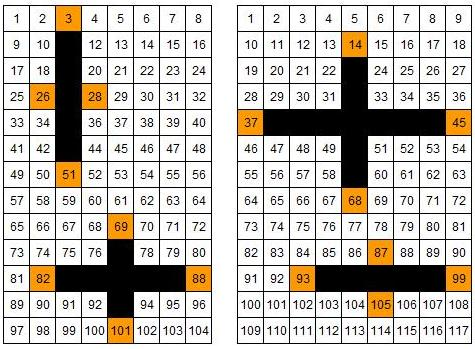### Submit a Resource

NRICH: Crossed Ends

Crosses can be drawn on number grids of various sizes. What do you notice when you add opposite ends?

These crosses can be drawn on number grids of various sizes.

Add opposite pairs of orange numbers
(i.e. north + south, east + west).

Notice anything?

Try a few more.Now try adding opposite pairs on crosses with just two lines of symmetry, like these:

What do you notice?

Experiment with grids of various sizes.

You may find these printable sheets useful:
Number Grids – width 3, 4, 5 and 6
Number Grids – width 7 and 8
Number Grids – width 9 and 10What happens if you add the orange squares in adjacent pairs? (try N + W, S + E )

Can you predict in advance how the totals will relate to each other?
What does it depend on?
Is it the same if you added them the other way round? (i.e. N + E, S + W)

Age 11 to 14

Math Topics
Algebra & Pre-Algebra, Linear Equations
Middle School, High School, Educator

## Organization

NRICH (University of Cambridge)

Challenge